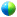19th Symposium on Boundary Layers and Turbulence

8.4

Impact of the lowest model layer height on performance of PBL parameterizations in numerical prediction

Hyeyum Hailey Shin, Yonsei University, Seoul, South Korea; and S. Y. Hong

This study investigates impact of the lowest model layer height on performance of five PBL parameterizations in the Weather Research and Forecasting (WRF) model for a day of the Cooperative Atmosphere-Surface Exchange Study (CASES-99) field program. The first model layer height is important because the height is assumed as the surface-layer depth where the surface layer assumption is applied. The five PBL parameterizations include two first-order closure models – the Yonsei University (YSU) PBL scheme and Asymmetric Convective Model version 2 (ACM2) – and three one-and-a-half-order closure (or TKE closure) models – the Mellor-Yamada-Janjić (MYJ), Quasi-Normal Scale Elimination (QNSE), and Bougeault-Lacarrére (BouLac) schemes.

To evaluate the impact of the lowest model layer height, four experiments with four different lowest model-layer heights (z1) are conducted for each PBL scheme: control, E978, E996, and E999 experiments. 1) In the control simulation, the lowest (first) and second full sigma levels are sigma1=0.990 and sigma2=0.978, respectively; the lowest half-sigma level height (z1) is about 40 m in the control simulation. 2) In the E978 setting, the lowest full sigma level is 0.978 (sigma1=0.978), which is corresponding to the second full sigma level of the control setting; the z1 is about 90 m. 3) In the E996 and E999 experiments, the lowest full sigma levels are sigma1=0.996 and sigma2=0.999 that are corresponding to z1 of 16 m and 4 m, respectively.

Preliminary results reveal that the simulated surface variables from the five PBL schemes (i.e., surface and 2-m temperatures, 10-m wind speed, surface friction velocity, surface sensible and latent heat fluxes) are significantly altered only when the lowest model layer height is too low as about several meters, during the daytime; the variables gradually approach observations by decreasing the height from O(100 m) to O(1 m) during the nighttime, except the YSU PBL scheme. Apropos of the vertical profiles, the YSU and ACM2 PBL parameterizations are sensitive to the surface layer height, and too shallow surface layer height about several meters (i.e., E999) always exhibits worsened predictability with these two parameterizations. On the other hand, the three TKE schemes – the MYJ, QNSE, and BouLac PBL schemes – are nearly insensitive to the surface layer height.Recorded presentation

Session 8, Boundary-layer Processes in Global and Regional Climate or Weather Prediction Models I
Thursday, 5 August 2010, 1:30 PM-3:00 PM, Torrey's Peak I&II

Previous paper  Next paper

Browse or search entire meeting